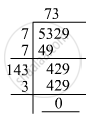Advertisement Remove all ads

# The Cost of Levelling and Turfing a Square Lawn at Rs 2.50 per M2 is Rs 13322.50. Find the Cost of Fencing It at Rs 5 per Metr - Mathematics

The cost of levelling and turfing a square lawn at Rs 2.50 per m2 is Rs 13322.50. Find the cost of fencing it at Rs 5 per metre.

Advertisement Remove all ads

#### Solution

First, we have to find the area of the square lawn, which the total cost divided by the cost of levelling and turfing per square metre:

Area of a square= 13322.5/2.5=5329 m^2

The length of one side of the square is equal to the square root of the area. We will use the long division method to find it as shown below:∴ Length of one side of the square = 73 m
The circumference of the square is 73 xx 4 = 292 m
∴ Total cost of fencing the lawn at Rs. 5 per metre = 292 xx 5 = Rs. 1460

Is there an error in this question or solution?
Advertisement Remove all ads

#### APPEARS IN

RD Sharma Class 8 Maths
Chapter 3 Squares and Square Roots
Exercise 3.5 | Q 10 | Page 44
Advertisement Remove all ads

#### Video TutorialsVIEW ALL 

Advertisement Remove all ads
Share
Notifications

View all notifications

Forgot password?
Course